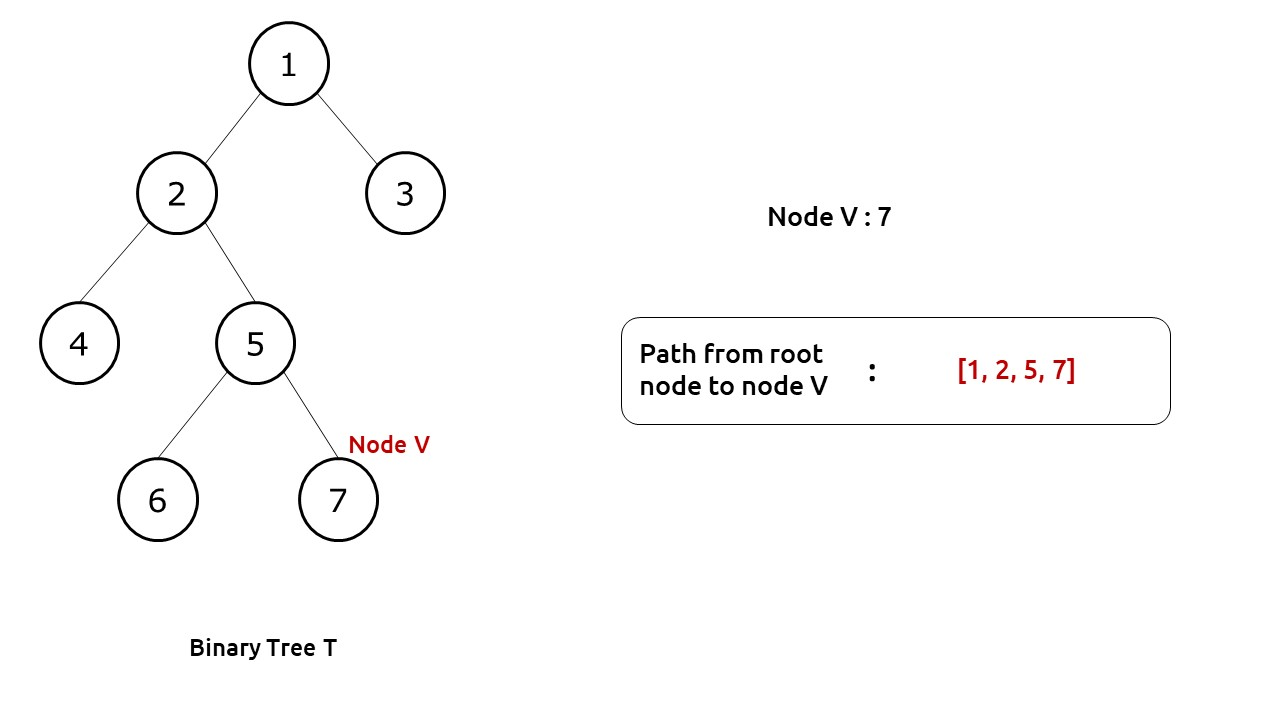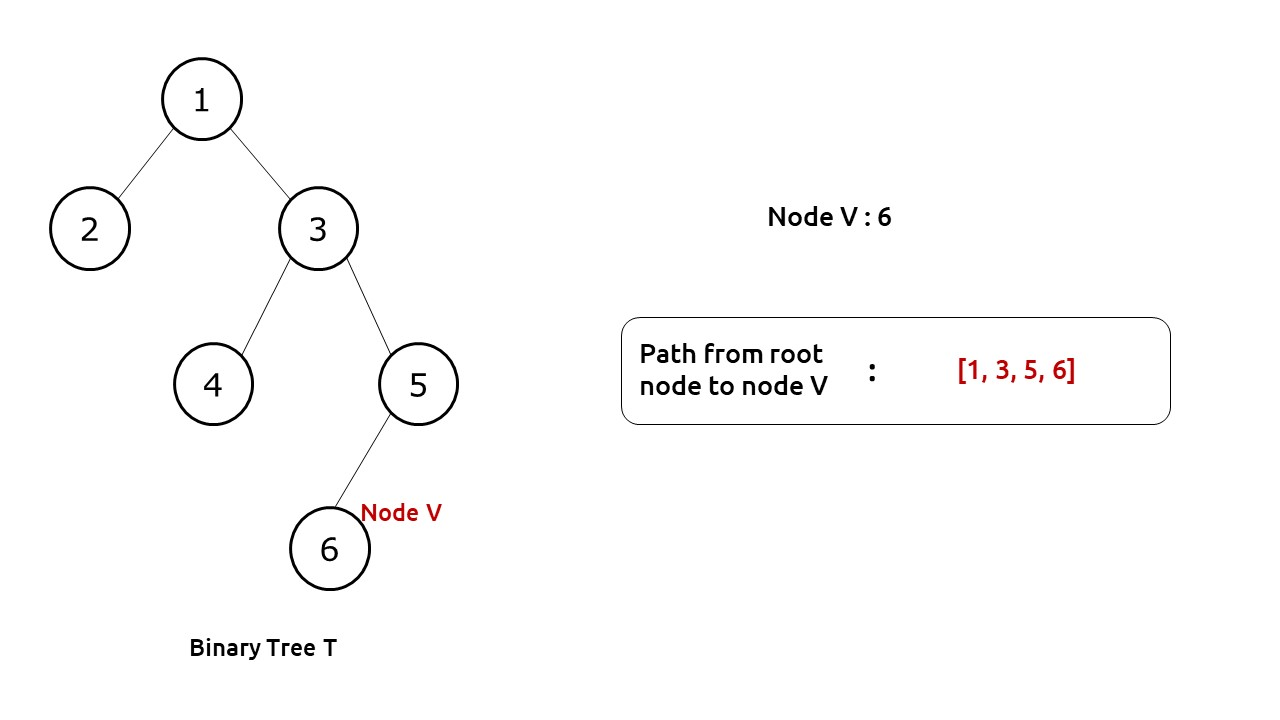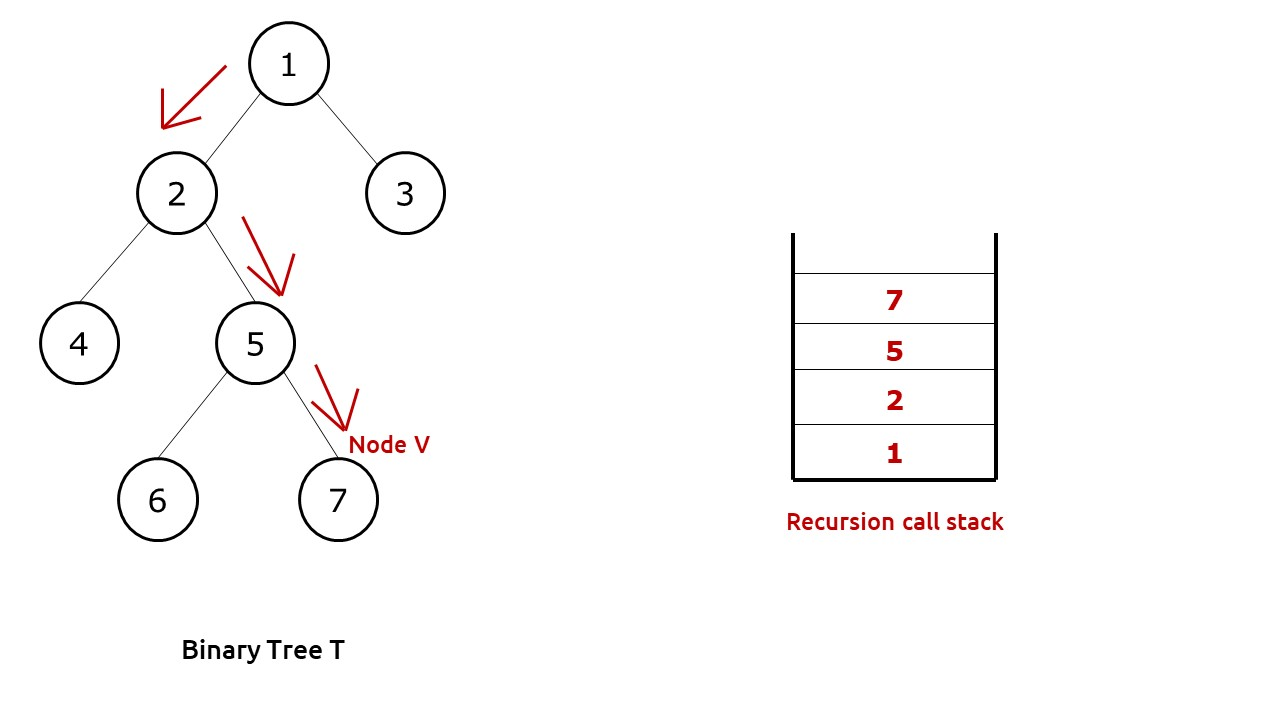# Print Root to Node Path in a Binary Tree

Problem Statement: Print Root to Node Path In A Binary Tree. Write a program to print path from root to a given node in a binary tree.

Problem Description:

We are given a binary tree T and a node V. We need to print a path from the root of the tree to the node.

Note:

• No two nodes in the tree have the same data value.
• It is assured that the node V is present and a path always exists.

Examples:

``
``

Disclaimer: Don’t jump directly to the solution, try it out yourself first.

Pre-req: Traversal Techniques & Recursion

Solution :

Intuition:

First of all, we need to find the node V in our tree for which we need to find the path. We can use any depth-first traversal technique (preorder, inorder, postorder) in order to find the required node.

If we look at the diagram below, we see that whenever we find the required node, its path is well present in our recursion call stack. We just need to figure out how we can use the recursive calls to print the required path.Approach:

We will use an external list to store our path. This list will be passed by reference to our recursive function. Moreover, we can set the return value of our function as boolean, this will help us to know whether node V was found in a subtree or not.

The algorithm steps can be stated as follows:

• We pass the function with our root node, the path list and node V.
• For the base case, if root is pointing to NULL, we return false as clearly node V can’t be found.
• Now we first push the node to our path list.
• Then we check whether the current node is the target node or not, if it is then no further execution is needed and we return to the parent function.
• If not, then we recursively call its left and right child to find the target node V. If any one of them returns true, it means we have found node V at lower levels and return true from the current function.
• If the value is not found at the current node and neither in any of the recursive calls, it means that the value is not present in the current sub-tree, therefore we pop out the current node from the path list and return false.

Dry Run: In case you want to watch the dry run for this approach, please watch the video attached below.

Code:

## C++ Code

``````#include <bits/stdc++.h>

using namespace std;

struct node {
int data;
struct node * left, * right;
};

bool getPath(node * root, vector < int > & arr, int x) {
// if root is NULL
// there is no path
if (!root)
return false;

// push the node's value in 'arr'
arr.push_back(root -> data);

// if it is the required node
// return true
if (root -> data == x)
return true;

// else check whether the required node lies
// in the left subtree or right subtree of
// the current node
if (getPath(root -> left, arr, x) ||
getPath(root -> right, arr, x))
return true;

// required node does not lie either in the
// left or right subtree of the current node
// Thus, remove current node's value from
// 'arr'and then return false
arr.pop_back();
return false;
}

struct node * newNode(int data) {
struct node * node = (struct node * ) malloc(sizeof(struct node));
node -> data = data;
node -> left = NULL;
node -> right = NULL;

return (node);
}

int main() {

struct node * root = newNode(1);
root -> left = newNode(2);
root -> left -> left = newNode(4);
root -> left -> right = newNode(5);
root -> left -> right -> left = newNode(6);
root -> left -> right -> right = newNode(7);
root -> right = newNode(3);

vector < int > arr;

bool res;
res = getPath(root, arr, 7);

cout << "The path is ";
for (auto it: arr) {
cout << it << " ";
}

return 0;
}``````

Output:

The path is 1 2 5 7

Time Complexity: O(N)

Reason: We are doing a simple tree traversal.

Space Complexity: O(N)

Reason: In the worst case (skewed tree), space complexity can be O(N).

## Java Code

``````import java.util.*;
class Node {
int data;
Node left, right;
Node(int data) {
this.data = data;
left = null;
right = null;
}
}
public class TUF {
static boolean getPath(Node root, ArrayList < Integer > arr, int x) {
// if root is NULL
// there is no path
if (root == null)
return false;

// push the node's value in 'arr'

// if it is the required node
// return true
if (root.data == x)
return true;

// else check whether the required node lies
// in the left subtree or right subtree of
// the current node
if (getPath(root.left, arr, x) ||
getPath(root.right, arr, x))
return true;

// required node does not lie either in the
// left or right subtree of the current node
// Thus, remove current node's value from
// 'arr'and then return false
arr.remove(arr.size() - 1);
return false;
}

public static void main(String args[]) {

Node root = new Node(1);
root.left = new Node(2);
root.left.left = new Node(4);
root.left.right = new Node(5);
root.left.right.left = new Node(6);
root.left.right.right = new Node(7);
root.right = new Node(3);

ArrayList < Integer > arr = new ArrayList < > ();

boolean res;
res = getPath(root, arr, 7);

System.out.print("The path is ");
for (int it: arr) {
System.out.print(it + " ");
}

}
}``````

Output:

The path is 1 2 5 7

Time Complexity: O(N)

Reason: We are doing a simple tree traversal.

Space Complexity: O(N)

Reason: In the worst case (skewed tree), space complexity can be O(N).

Special thanks to Anshuman Sharma for contributing to this article on takeUforward. If you also wish to share your knowledge with the takeUforward fam, please check out this article